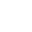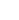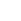Make compact charts inside worksheet cells using Excel's REPT() function and Excel's conditional formatting. Download a copy of the worksheet here. Discuss this video in the forum.

These videos are best viewed in full screen mode. Press play and double click the screen to maximise.

How to make Gannt charts using the Excel REPT() function. Download the Gannt chart here. Discuss this video in the forum.

These videos are best viewed in full screen mode. Press play and double click the screen to maximise.

When you add a trendline to a chart, Excel provides an option to display the trendline equation in the chart. This tip describes how to create formulas that generate the trendline coefficients. You can then use these formulas to calculate predicted y values for give values of x.

1) First the Easy Way

These videos are best viewed in full screen mode. Press play and double click the screen to maximise.

2) Now the More Complex 'Dynamic' Way

These videos are best viewed in full screen mode. Press play and double click the screen to maximise.

The following cell formulae will dynamically calculate trendline equation parameters from a data table. The trendline formulae are quite complicated so copy and paste into your worksheet. These equations assume that your sheet contains C5:H5 (known_x's) and C8:H8 (known_y's).

Linear Trendline

Equation: y = m * x + b
m: =SLOPE(C8:H8,C5:H5)
b: =INTERCEPT(C8:H8,C5:H5)

Logarithmic Trendline
Equation: y = (c * LN(x)) + b
c: =INDEX(LINEST(C8:H8,LN(C5:H5)),1)
b: =INDEX(LINEST(C8:H8,LN(C5:H5)),1,2)

Power Trendline
Equation: y=c*x^b
c: =EXP(INDEX(LINEST(LN(C8:H8),LN(C5:H5),,),1,2))
b: =INDEX(LINEST(LN(C8:H8),LN(C5:H5),,),1)

Exponential Trendline
Equation: y = c *e ^(b * x)
c: =EC5:H5P(INDEX(LINEST(LN(C8:H8),C5:H5),1,2))
b: =INDEX(LINEST(LN(C8:H8),C5:H5),1)

2nd Order Polynomial Trendline
Equation: y = (c2 * x^2) + (c1 * x ^1) + b
c2: =INDEX(LINEST(C8:H8,C5:H5^{1,2}),1)
C1: =INDEX(LINEST(C8:H8,C5:H5^{1,2}),1,2)
b = =INDEX(LINEST(C8:H8,C5:H5^{1,2}),1,3)

3rd Order Polynomial Trendline
Equation: y = (c3 * x^3) + (c2 * x^2) + (c1 * x^1) + b
c3: =INDEX(LINEST(C8:H8,C5:H5^{1,2,3}),1)
c2: =INDEX(LINEST(C8:H8,C5:H5^{1,2,3}),1,2)
C1: =INDEX(LINEST(C8:H8,C5:H5^{1,2,3}),1,3)
b: =INDEX(LINEST(C8:H8,C5:H5^{1,2,3}),1,4)

Higher Order Polynomial Trendline
Follow the pattern set in the second and third order polynomial trendlines.

In the Forum

Some Sample Calculations on the SitePurpose of calculation: Calculate stress in a 'semi circular' tension fitting (or bath tub fitting).

###Angle type tension fitting.xlsPurpose of calculation: Calculate stress in a 'angle' tension fitting...

###Channel type tension fitting.xlsPurpose of calculation: Calculate stress in a 'channel' tension fitting...XLC has some special functions to control what will displayed OVer the Division line, OVD(), and UNder the Division line, UND(). E.g. the formula "=OVD(1)/UND(2)" will be displayed in fractional form. Using XLC you are likely to type the construct "OVD()/UND()" many times. Use the “Copy OVD()/UND() to clipboard” button and paste into a cell formula using the CTRL+V shortcut. It's a simple but effective time saver.

 Play movie - Copy OVD()/UND() A simple tool to save time typing XLC OVD()/UND() functions.

What does OVD()/UND() do?Notes

Failure to position the functions in this order OVD()/UND() will result in the following error "#OVD()/UND() function misuse. Check that OVD() occurs immediately before / and UND() occurs immediately after /.#"

OVD()/UND() functions cannot be nested.

German Version of Excel

UND() is used as the logical AND() function in German versions of MS Excel. To prevent conflicts:

UDI() can be optionally used in place of UND().

and for the sake of consistency ODI() can be optionally used in place of OVD().Greek characters are used extensively in equations and formulae. XLC makes it easy to access Greek characters using typing shortcuts. XLC will process any character preceded by a “|” character by converting it into a Greek equivalent. For example type “|a” to obtain “α”.If you are not too familiar with the Greek alphabet use the “Show Greek character shortcut” button on the XLC toolbar.The “Toggle auto/equal chart XYscales”. Use this button with Excel scatter charts to produce drawings or sketches. If you use scatter charts to draw a square shape using co-ordinates Excel will automatically scale the x and y scales to best fit the chart area but this is likely to mean that you see a rectangular shape rather than a square shape. Select the chart and use this button to make the x scale equal to the Y scale and you can see the square. Use the button again and the X and Y scale will be automatically scaled. It’s very useful.Play movie - Sketch using scatter charts Learn how to automatically adjust scatter chart X and Y scales so that sketches are correctly scaled.